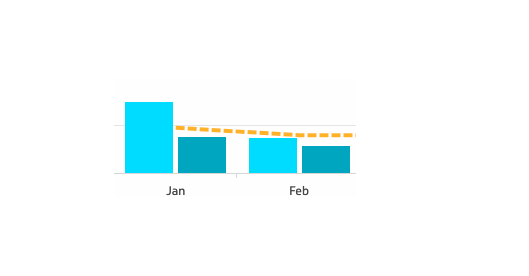Hello Klipfolio Community!

I am trying to calculate a running total in over several months through to TODAY(). For example if TODAY() is March 31st  and the following values represented widgets sold in each month:
jan = 100
feb = 200
mar = 50

I would want a bar or line graph that shows the following running total values:
running total
jan = 100
feb = 300
mar = 350

Then I would like to show how this running total lines up with a goal for example if the goal = 500 then I could show that:
jan is 20% to goal
feb is 60% to goal
mar is 70% to goal

Either by providing another line on the chart to show  on a different axis percentages or by including a data label that shows the percentage to goal.

So far what I have is a monthly value and a goal by month represented by the yellow dotted line#### 1 comment

•Keely Davison

Hi Ashley,

Great question.  For the cumulative series you can use the CUMULATIVE function.

e.g. CUMULATIVE(@A:A)

There are a couple of caveats to this.  If you are grouping or sorting on your x-axis you made need a couple of other steps. Let us know if this is the case.

If the goal is a 2nd series as you have shown you can use results references to the other series to get the percent.

e.g. &actual /&goal *100

Set the format for this series to be percentage and I agree with you that I would make a secondary y-axis and associate this series with it.

If the goal is a single value you can use results references for actuals with the goal value as follows

e.g. &actual/500 *100

Here is some information on the cumulative function and results references.

https://support.klipfolio.com/hc/en-us/articles/360011416893-Klipfolio-functions#CUMULATIVE

https://support.klipfolio.com/hc/en-us/articles/115000181613-Writing-formulas-using-results-references

Keely